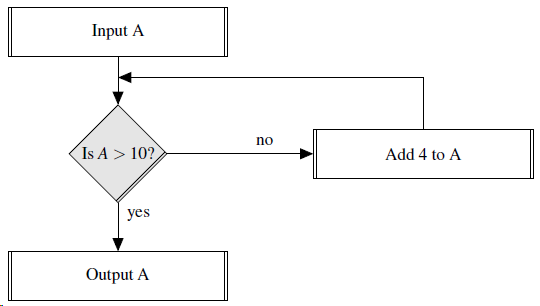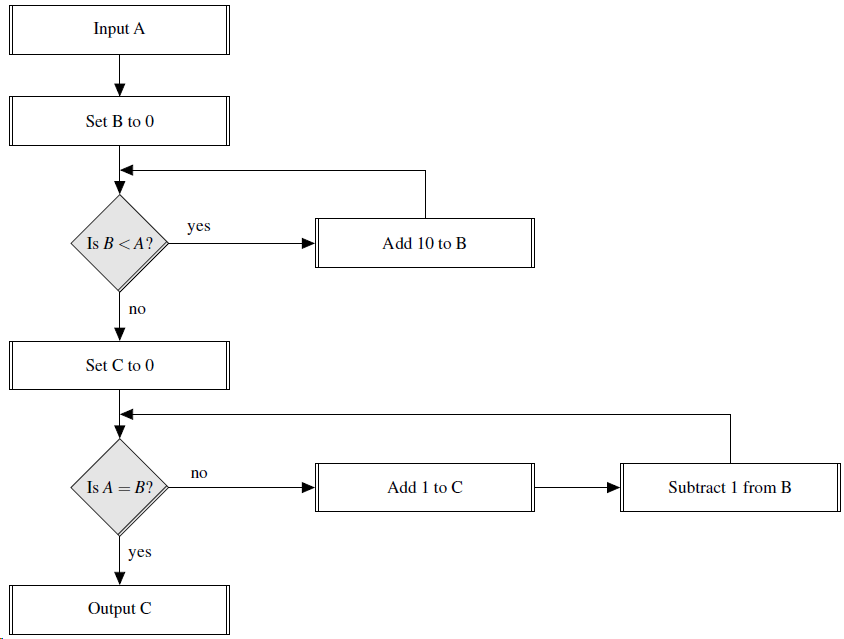Flow diagrams provide a visual way of showing a process or algorithm: a box is used for an action, a diamond (shaded) for making a decision, and arrows indicate the flow of control.

For example, in this simple flow diagram, if A was input as 9 it would be output as 13, whilst if it was input as 11 it would be unchanged and output as 11.Question:

For the flow diagramwhat is the output if the input is 36?